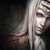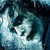24,360 Pages

## Introduction

In physics, the kinetic energy of an object is the energy that it possesses due to its motion. It is defined as the work needed to accelerate a body of a given mass from rest to its stated velocity. Having gained this energy during its acceleration, the body maintains this kinetic energy unless its speed changes. The same amount of work is done by the body in decelerating from its current speed to a state of rest.

In classical mechanics, the kinetic energy of a non-rotating object of mass m travelling at a speed v is $\tfrac{1}{2}mv^2$.

## Rules

Kinetic energy of objects can be used for various calculations.

However the following rules apply:

• No kinetic energy for faster than light speeds: Kinetic energy calculated for faster than light objects, or more precise for objects for which $v \ge 299 792 458 m / s$, is not considered legitimate. That is because the kinetic energy of an object, using the correct physical description through relativistic mechanics, would require infinite energy to reach the speed of light, and for objects above the speed of light the equations wouldn't return real values. Since the energy would approach infinity towards the speed of light it also isn't allowed to use relativistic speed as an approximation for the kinetic energy of faster than light objects, since by using an approximation close enough to the speed of light any given value could be reached through that method.
• Do not calculate speed from kinetic energy: The kinetic energy an object was calculated to possess, in any way whatsoever, should not be considered as related through its speed. While the formula technically can be used to relate those values in both direction this is disregarded in practice. One reason for this is that fiction in general differentiates between the attack potency and the speed of a character. Another reason is that it returns unrealistic values, as even a Small City level+ punch would already have Relativistic+ speed. Out of similar reasons mass should also not be calculated from it.
• The relativistic kinetic energy value is only accepted up to 4 times the Newtonian value: The kinetic energy value calculated using the formula for relativistic kinetic energy is only accepted to the point where it is 4 times as high as the value of Newtonian kinetic energy. That is the case, if the speed of the moving object is above 93% of the speed of light. For kinetic energy values above that, which are not faster than light, 4 times the kinetic energy value should be taken. Reason for this rule is that the relativistic kinetic energy diverges towards infinity for speeds approaching the speed of light. So to not get inflated extremely high results setting a threshold at 4 times the kinetic energy value was decided upon.
An exception to this rule would be if a specific value is explicitly stated: For example, the Flash hitting with the force of a white dwarf star.
• Kinetic Energy based on Movement Speed is case by case: Fiction often treats the speed with which a character can move himself as unrelated to their attack power. As such feats like just running or carrying a small object, like another character, should only be used if the fiction has made clear that the speed of the movement correlates to the character's power or if the character uses the fast moving object to attack. Calculating the energy necessary for moving large structures at great speeds, using the speed things move as a secondary effect of an attack, throwing objects at great speeds etc. are all acceptable methods of quantifying a characters power regardless.

## Speed can be used to find KE when

• A certain character moves at a certain speed while carrying an object, that would require superhuman strength to be carried.
• The kinetic energy displayed is directly shown to be as a result of speed, or at least heavily implied to be so. Examples would be: A meteor crashing into the ground, as well as a Kaiju moving at full speed.
• A verse consistently treats speed, and its relation to attack potency, in a realistic manner. Calculating kinetic energy from a character's speed isn't problematic, if it's clear that the story they're from doesn't separate speed from one's general capacity for destruction.
• A projectile has been calculated to move at a certain speed, such as a cannonball or a spear.
• An object moves at said speed due to the secondary effects of an attack. For example when an explosion tosses large rocks around, their KE can be used to measure the power of the explosion.
• A feat happens in the Real World, since physics work normally in real life.

## Speed cannot be used to find KE when

• The calculated kinetic energy value is heavily inconsistent with the rest of the cast in the series. EX: Quicksilver's calculated speed cannot be used to derive kinetic energy as it heavily contradicts his established power levels.
• There is a destruction/AP calculation contradicting a kinetic energy calculation. The destruction/AP calculation would take priority over the kinetic energy calculation in this case as the AP calculation would be a better proof in regards to how much damage he/she is capable of in an attack.
• For example, if a character launches a 200kg metal ball against a common wall at Mach 300, but the wall remains largely undamaged, the energy required to cause the minor damage on the wall would take priority over the kinetic energy derived from speed in this case.

## Start a Discussion Discussions about Kinetic Energy Feats

•#### How great does a kinetic energy feat have to be, to be an outlier?

•A lot of the time when i see characters ranked at a certain level for a kinetic energy feat, their tiers are often vastly above any other non...
•#### Can Reaction Speed be used to find KE?

15 messages
•@MJF https://vsbattles.fandom.com/wiki/Message_Wall:Christian_Higdon created the page.
•I guess I agree, but I've had enough taking shit.
Community content is available under CC-BY-SA unless otherwise noted.